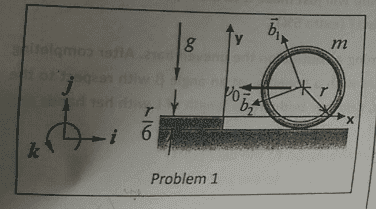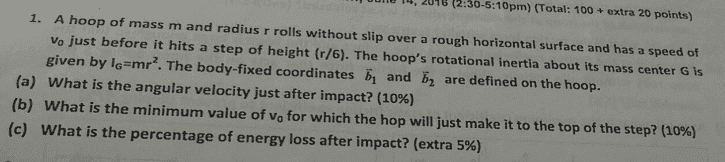# Minimum Vo for a hoop to get to the top

Cleo James

## Homework Statementpart(b) and (c)

## Homework Equations

Conservation of momentum
Conservation of angular momentum
Conservation of mechanical energy

## The Attempt at a Solution

So first I thought that I could do it by just using the conservation of mechanical energy, but then I realized that since the lower part of the hoop hits the platform, the energy can't be conserved (there's energy lost):

1/2 mv^2 + 1/2 Iw^2 = mgh + Energy lost
mv^2 = mgh + Energy lost

I think that part (b) and (c) is very related, once we know the energy lost, we can determine the v needed, however I don't really understand how to find its value. Any suggestion ??

Homework Helper
Gold Member
You are right that KE will not be conserved. What other conservation possibilities are there?
You mentioned two. What is stopping you from trying to use them?

Last edited:
Cleo James
You are right that KE will not be conserved. What other conservation possibilities are there?
You mentioned two. What is stopping you from trying to use them?
Well, conservation of momentum:
m1v1 + m2v2 = (m1+m2)v3
But this is if the object experiences inelastic collision, I don't know how to apply it when the other object is just a small bump.
Conservation of angular momentum:
Iw1= Iw2
since the hoop will rotate about the edge of the bump during collision, parallel axis theorem:
mr^2 w1= 2mr^2 w2
w1= 2w2

At this time I can't seem to figure out what to do afterwards.

Homework Helper
Gold Member
Well, conservation of momentum:
m1v1 + m2v2 = (m1+m2)v3
But this is if the object experiences inelastic collision, I don't know how to apply it when the other object is just a small bump.
Conservation of angular momentum:
Iw1= Iw2
since the hoop will rotate about the edge of the bump during collision, parallel axis theorem:
mr^2 w1= 2mr^2 w2
w1= 2w2

At this time I can't seem to figure out what to do afterwards.
The problem with those two conservation laws here is that you have an unknown impulse from the step. So you need to find a way of applying one of them in which that impulse makes no contribution.
If you knew the direction of the impulse you could consider momentum orthogonal to it; but you don't.
Angular momentum is always relative to some chosen axis. Can you choose an axis such that the impulse has no moment about it?